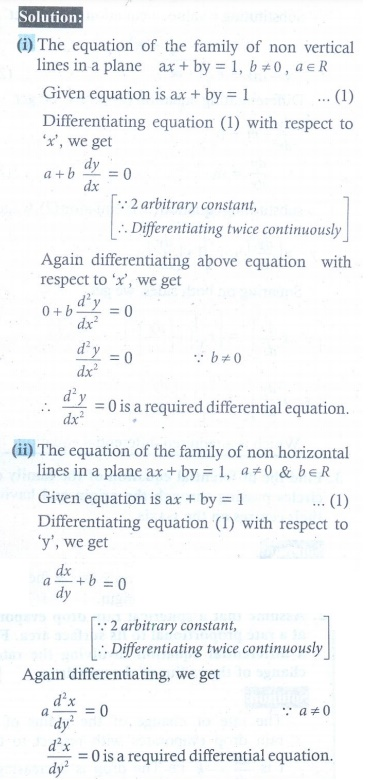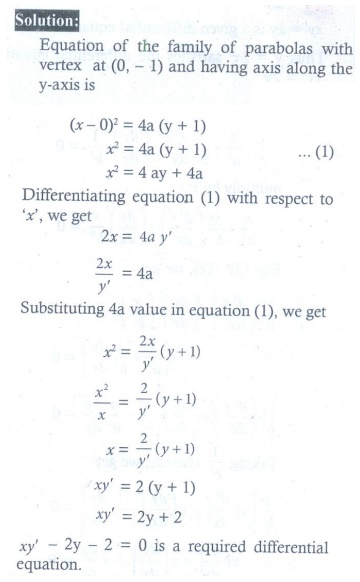Home | | Maths 12th Std | Exercise 10.3: Formation of Differential Equations from Geometrical Problems

# Exercise 10.3: Formation of Differential Equations from Geometrical Problems

Maths Book back answers and solution for Exercise questions - Mathematics : Ordinary Differential Equations: Formation of Differential Equations from Geometrical Problems - Exercise Problem Questions with Answer, Solution

EXERCISE 10.3

1. Find the differential equation of the family of (i) all non-vertical lines in a plane (ii) all non-horizontal lines in a plane.2. Form the differential equation of all straight lines touching the circle x2 + y2 = r2.3. Find the differential equation of the family of circles passing through the origin and having their centres on the x -axis.4. Find the differential equation of the family of all the parabolas with latus rectum 4a and whose axes are parallel to the x -axis.5. Find the differential equation of the family of parabolas with vertex at (0, 1) and having axis along the y -axis.6. Find the differential equations of the family of all the ellipses having foci on the y -axis and centre at the origin.7. Find the differential equation corresponding to the family of curves represented by the equation y = Ae8x + Be8x , where A and B are arbitrary constants.8. Find the differential equation of the curve represented by xy = ae x + be  x + x2.3. x2 + 2xy dy/dx − y2 = 0

4. 2ay ′′ + y3 = 0

5.  xy′ − 2− 2 = 0

6. xy ′ 2 + xyy′′ − yy′ = 0

7. d2y/dx = 64 y

8. xy′′ + 2 y ′ + x2 − xy − 2 = 0

Tags : Problem Questions with Answer, Solution , 12th Maths : UNIT 10 : Ordinary Differential Equations
Study Material, Lecturing Notes, Assignment, Reference, Wiki description explanation, brief detail
12th Maths : UNIT 10 : Ordinary Differential Equations : Exercise 10.3: Formation of Differential Equations from Geometrical Problems | Problem Questions with Answer, Solution